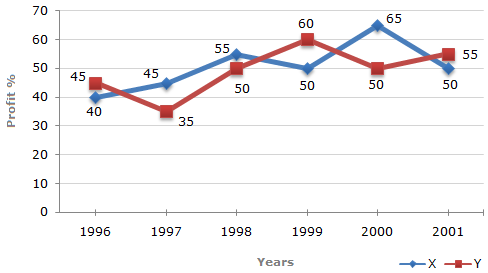# Data Interpretation - Line Charts

Exercise : Line Charts - Line Chart 4
Directions to Solve

The following line graph gives the percent profit earned by two Companies X and Y during the period 1996 - 2001.

Percentage profit earned by Two Companies X and Y over the Given Years

 %Profit = Income - Expenditure x 100 Expenditure1.
The incomes of two Companies X and Y in 2000 were in the ratio of 3:4 respectively. What was the respective ratio of their expenditures in 2000 ?
7:22
14:19
15:22
27:35
Explanation:

Let the incomes in 2000 of Companies X and Y be 3x and 4x respectively.

And let the expenditures in 2000 of Companies X and Y be E1 and E2 respectively.

Then, for Company X we have:

 65 = 3x - E1 x 10065 = 3x - 1E1 = 3x x100.... (i) E1 100 E1 165

For Company Y we have:

 50 = 4x - E2 x 10050 = 4x - 1E2 = 4x x100.... (ii) E2 100 E2 150

From (i) and (ii), we get:

E1 =
 3x x100165
= 3 x 150 = 15 (Required ratio).
E2
 4x x100150
4 x 165 22

2.
If the expenditure of Company Y in 1997 was Rs. 220 crores, what was its income in 1997 ?
Rs. 312 crores
Rs. 297 crores
Rs. 283 crores
Rs. 275 crores
Explanation:

Profit percent of Company Y in 1997 = 35.

Let the income of Company Y in 1997 be Rs. x crores.

 Then, 35 = x - 220 x 100x = 297. 220Income of Company Y in 1997 = Rs. 297 crores.

3.
If the expenditures of Company X and Y in 1996 were equal and the total income of the two Companies in 1996 was Rs. 342 crores, what was the total profit of the two Companies together in 1996 ? (Profit = Income - Expenditure)
Rs. 240 crores
Rs. 171 crores
Rs. 120 crores
Rs. 102 crores
Explanation:

Let the expenditures of each companies X and Y in 1996 be Rs. x crores.

And let the income of Company X in 1996 be Rs. z crores.

So that the income of Company Y in 1996 = Rs. (342 - z) crores.

Then, for Company X we have:

 40 = z - x x 10040 = z - 1x = 100z .... (i) x 100 x 140

Also, for Company Y we have:

 45 = (342 - z) x 10045 = (342 - z) - 1x = (342 - z) x 100 .... (ii) x 100 x 145

From (i) and (ii), we get:

 100z = (342 - z) x 100z = 168. 140 145

Substituting z = 168 in (i), we get : x = 120.Total expenditure of Companies X and Y in 1996 = 2x = Rs. 240 crores.

Total income of Companies X and Y in 1996 = Rs. 342 crores.Total profit = Rs. (342 - 240) crores = Rs. 102 crores.

4.
The expenditure of Company X in the year 1998 was Rs. 200 crores and the income of company X in 1998 was the same as its expenditure in 2001. The income of Company X in 2001 was ?
Rs. 465 crores
Rs. 385 crores
Rs. 335 crores
Rs. 295 crores
Explanation:

Let the income of Company X in 1998 be Rs. x crores.

 Then, 55 = x - 200 x 100x = 310. 200Expenditure of Company X in 2001 = Income of Company X in 1998 = Rs. 310 crores.

Let the income of Company X in 2001 be Rs. z crores.

 Then, 50 = z - 310 x 100z = 465. 310Income of Company X in 2001 = Rs. 465 crores.

5.
If the incomes of two Comapanies were equal in 1999, then what was the ratio of expenditure of Company X to that of Company Y in 1999 ?
6:5
5:6
11:6
16:15
Explanation:

Let the incomes of each of the two Companies X and Y in 1999 be Rs. x.

And let the expenditures of Companies X and Y in 1999 be E1 and E2 respectively.

Then, for Company X we have:

 50 = x - E1 x 10050 = x - 1x = 150 E1 .... (i) E1 100 E1 100

Also, for Company Y we have:

 60 = x - E2 x 10060 = x - 1x = 160 E2 .... (ii) E2 100 E2 100

From (i) and (ii), we get:

 150 E1 = 160 E2E1 = 160 = 16 (Required ratio). 100 100 E2 150 15